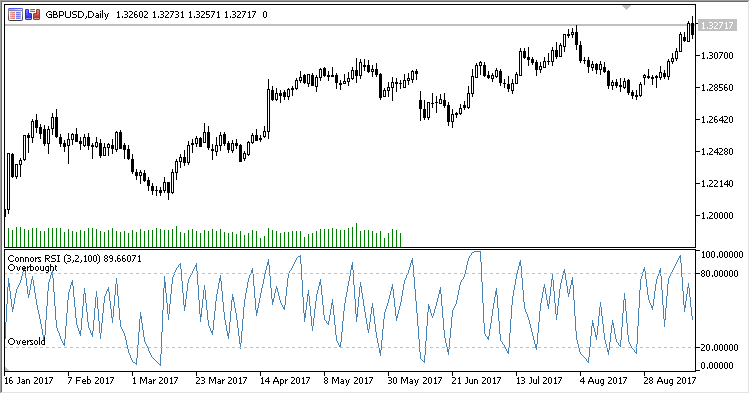|

1263

2018.11.08 08:58

Connors RSI 是由 Larry Connors 开发的 RSI 振荡指标。

• RSI period - 计算周期数
• Pulse RCI period - 波动 RSI 周期数
• Percent rank period - 计算变化率范围百分比
• Applied price
• Overbought - 超买水平
• Oversold - 超卖水平

```CRSI = (RSI1+RSI2+RSI3) / 3.0
```

```RSI1 - RSI(Applied price,RSI period)
RSI2 - RSI(UpDown,Smoothing period)
RSI3 - 100.0 * Count / Percent rank period
UpDown - 收盘价连续高于/低于前一天的天数:

Count - 两周期 RSI 在百分比周期数范围内的正向增加次数
```Simple_Decycler

Simple Decycler 指标Decycler_Oscillator

Decycler 振荡指标Avg_MAMA_Trend

MA 趋势指标